# 100 nm bandwidth flat-gain Raman amplifier – Average power model

Compatibility:

This lesson shows the performance of the Average power model in analysis of the 100 nm bandwidth Raman amplifier with multiwavelength backward pump. The parameters considered are close to these used in the experiment of . The same experimental situation has been modeled by means of Average power model in .

As is well known one of the largest challenges for the Raman amplifiers is to overcome the bandwidth limitations of EDFA. The above referenced experimental paper shows the possibility to perform gain-equalized Raman gain over 100 nm using a proper asymmetric channel allocations and powers of the pumps.

Our project is shown in Figure 1.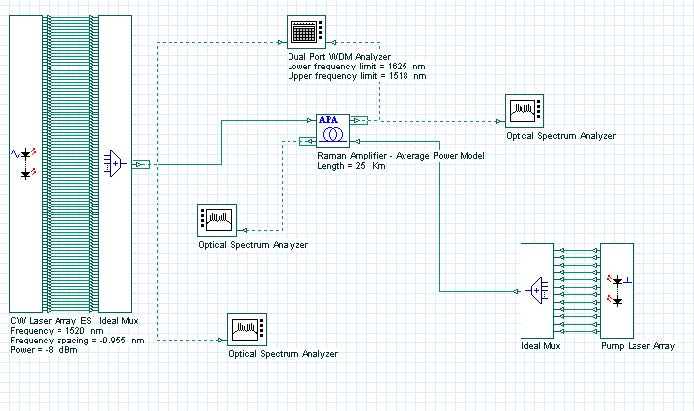Figure 1: Project Layout for analysis of the Raman gain

Following the data of , the spectral location and the magnitudes of the twelve backward pumps are arranged between 1405 and 1510 nm. The exact values for the locations and power of the pumps are shown in Figure 2. The pump powers are different than those presented in .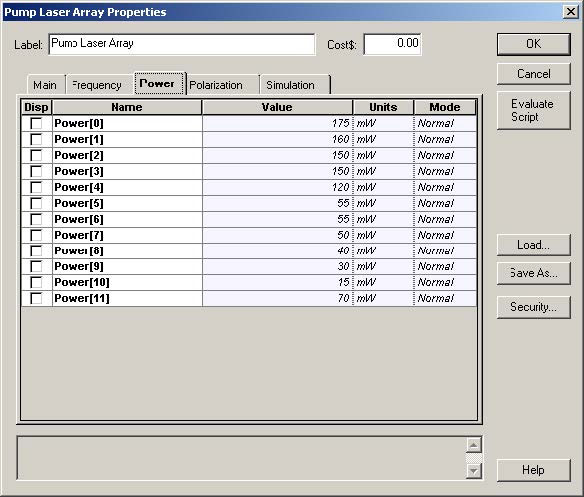Figure 2: Backward pumps locations and powers

Signals in 100 channels are from 1520 to approximately 1620 nm with spacing equal to 0.955 nm. The power is set to -8 dBm power. For generation of these signals we use a laser array with the above parameters.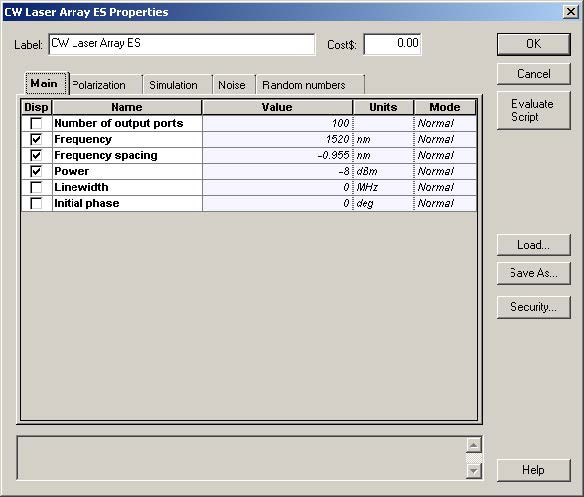Figure 3: 100 nm bandwidth signals

The amplifier has following parameters: length L = 25 km, amplifier absolute temperature = 300 K, polarization factor = 2. The background loss and the Raman gain efficiency are defined through files. These parameters are introduced in the tabs of the Raman component: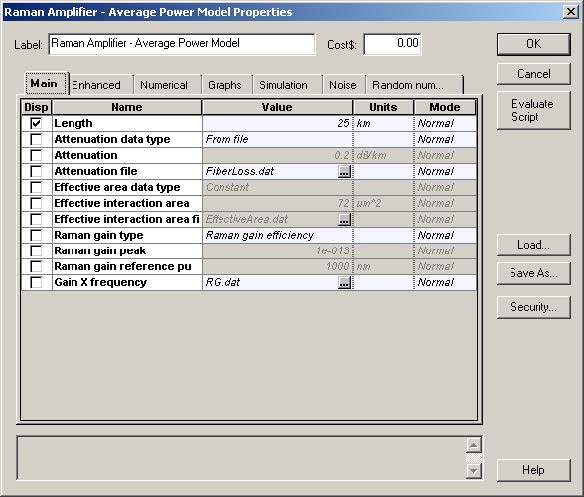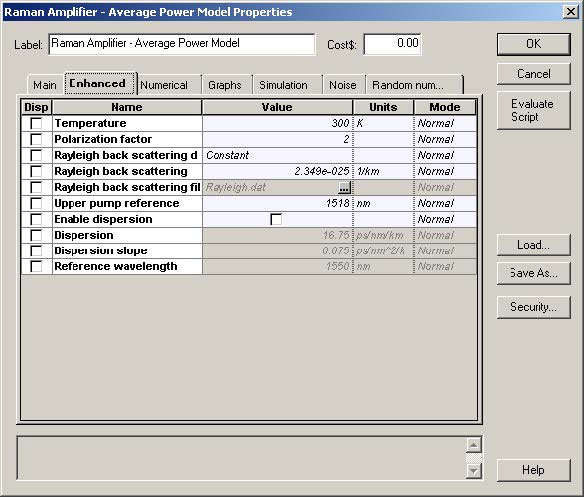Figure 4: Amplifier parameters

As we can see, when the gain type is set to “Raman gain efficiency”, the effective area and Raman peak are disabled. The upper pump reference is set to 1518 nm, after the amplifier is pumped up to 1510 nm and the signals start from 1520 nm.

The tab “Numerical” is used to set the numerical parameters for the calculation. The number of amplifier sections is set to 50 and convergence will be checked using all the signals.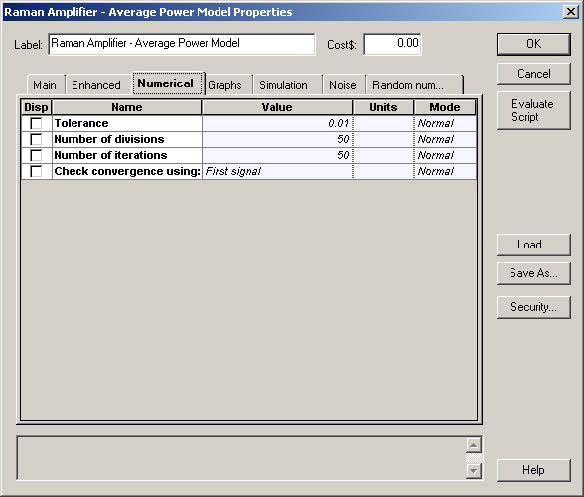Figure 5: Numerical settings

The description of ASE is given in Figure 6.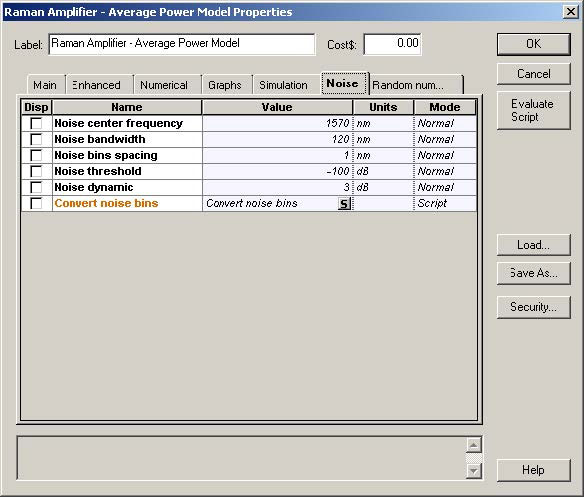Figure 6: Description of ASE

Note that the noise bins cover all the signal area and that the spacing between them is the same of the channels being transmitted that are illustrated on the next figure.

For such parameters the obtained gain for all the channels is shown in Figure 7.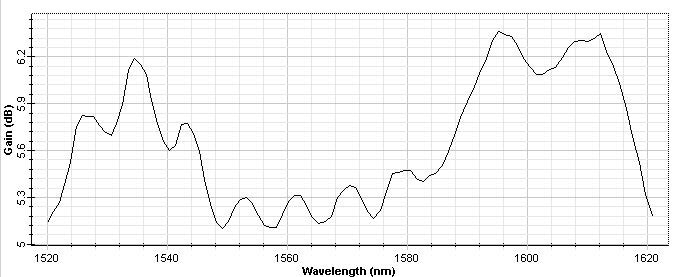Figure 7: Gain for the layout described above

This figure has to be compared with Figure 2 of .

The next phenomenon analyzed, is pump depletions due to Raman energy transfer that can be seen on the next figure. The qualitative picture of the energy transfers between the lower wavelengths to the higher ones is very well demonstrated in Figure 8.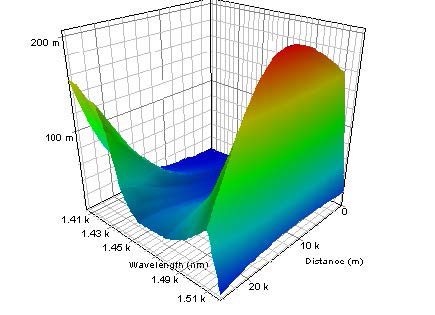Figure 8: Counter-propagating pump

In this lesson we have shown the performance of the Average power model for description of the 100 nm bandwidth flat-gain Raman amplifiers.

References:

 Y. Emori, K. Tanaka, and S. Namiki, “100 nm bandwidth flat-gain Raman amplifiers pumped and gain -equalised by 12-wavelength-channel WDM laser diode unit”, Electronics Letters, Vol. 35, No. 16, pp. 1355-1356, 1999.

 B. Min, W. J. Lee, N. Park, “Efficient Formulation of Raman Amplifier Propagation Equations with Average Power Analysis”, IEEE Photonics Technology Letters, Vol. 12, No. 11, November 2000.